WEIGHT Statement
WEIGHT variable ;

The WEIGHT statement specifies a numeric variable in the input data set with values that are used to weight each observation. Only one variable can be specified.

The WEIGHT variable values can be nonintegers. An observation is used in the analysis only if the value of the WEIGHT variable is greater than zero.

The WEIGHT variable applies only when you specify the following standardization methods: AGK, EUCLEN, IQR, L, MAD, MEAN, MEDIAN, STD, SUM, and USTD. Weights are used for the METHOD=MAD, MEDIAN, or IQR only when PCTLMTD=ORD_STAT is specified; if PCTLMTD=ONEPASS is specified, the WEIGHT statement is ignored.

PROC STDIZE uses the value of the WEIGHT variable to calculate the sample mean and sample variances: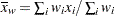(sample mean)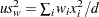(uncorrected sample variances)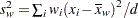(sample variances)

where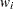is the weight value of the ith observation,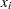is the value of the ith observation, and d is the divisor controlled by the VARDEF= option (see the VARDEF= option for details).

The following weighted statistics are defined accordingly:

MEAN

the weighted mean,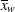SUM

the weighted sum,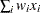USTD

the weighted uncorrected standard deviation,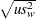STD

the weighted standard deviation,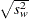EUCLEN

the weighted Euclidean length, computed as the square root of the weighted uncorrected sum of squares: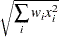MEDIAN

the weighted median. See the section Weighted Percentiles for the formulas and descriptions.

the weighted median absolute deviation from the weighted median. See the section Weighted Percentiles for the formulas and descriptions.

IQR

the weighted median, 25th percentile, and the 75th percentile. See the section Weighted Percentiles for the formulas and descriptions.

AGK

the AGK estimate. This estimate is documented further in the ACECLUS procedure as the METHOD=COUNT option. See the discussion of the WEIGHT statement in Chapter 23, The ACECLUS Procedure, for information about how the WEIGHT variable is applied to the AGK estimate.

L

the
estimate. This estimate is documented further in the FASTCLUS procedure as the LEAST= option. See the discussion of the WEIGHT statement in Chapter 35, The FASTCLUS Procedure, for information about how the WEIGHT variable is used to compute weighted cluster means. The number of clusters is always 1.